# Array.push() Element if does not exist using JavaScriptLast updated: Jan 10, 2023
5 min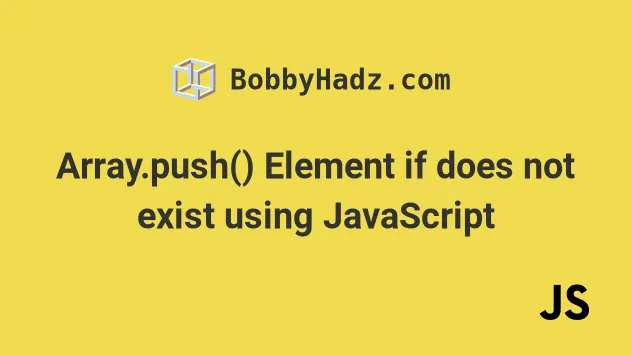## #Array.push() Element if does not exist in JavaScript

To push an element into an array if it doesn't exist:

1. Use the `Array.includes()` method to check if the value exists in the array.
2. Use the `Array.push()` method to push the value into the array if it doesn't already exist.
index.js
```Copied!```// ✅ With primitives
const arr = ['a', 'b', 'c', 'd'];
const value1 = 'e';

if (!arr.includes(value1)) {
arr.push(value1);
}

// 👇️ [ 'a', 'b', 'c', 'd', 'e' ]
console.log(arr);
``````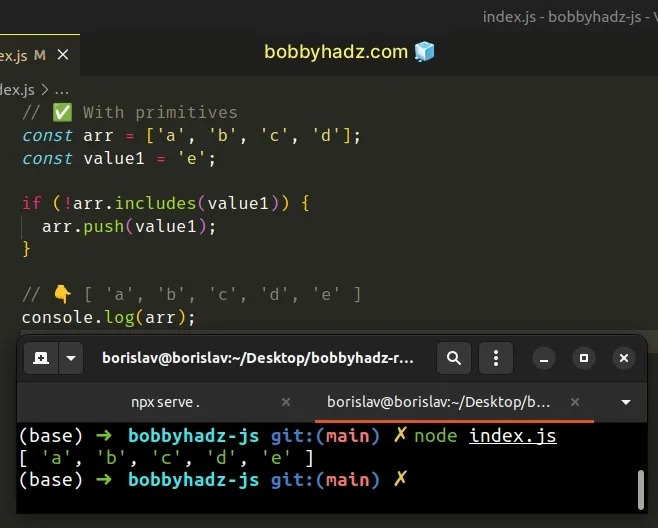If you have an array of objects, you have to use the Array.findIndex() method.

index.js
```Copied!```// ✅ With Objects
const arr = [{id: 1}, {id: 2}];
const value = {id: 3};

const index = arr.findIndex(object => object.id === value.id);

if (index === -1) {
arr.push(value);
}

// 👇️ [ { id: 1 }, { id: 2 }, { id: 3 } ]
console.log(arr);
``````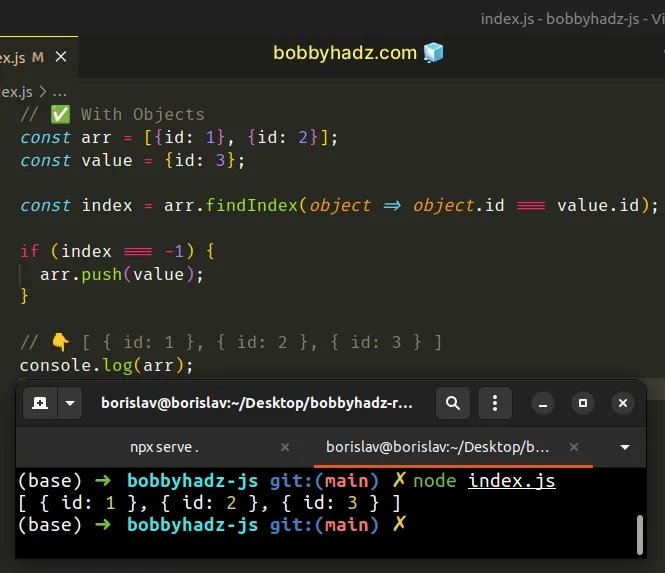The array in the first example contains primitive values (strings).

We used the logical NOT (!) operator to negate the call to the Array.includes() method.

index.js
```Copied!```const arr = ['a', 'b', 'c', 'd'];
const value = 'e';

console.log(arr.includes(value)); // 👉️ false

console.log(!arr.includes(value)); // 👉️ true
``````

The `Array.includes()` method returns `true` if the supplied value is contained in the array and `false` otherwise.

If the value isn't in the array, we use the `Array.push()` method to add it.

index.js
```Copied!```const arr = ['a', 'b', 'c', 'd'];
const value = 'e';

if (!arr.includes(value)) {
arr.push(value);
}

// 👇️ [ 'a', 'b', 'c', 'd', 'e' ]
console.log(arr);
``````

If you need to push an object into an array if it doesn't exist, use the `findIndex` method.

## #Array.push() Element if does not exist using `findIndex`

To push an object into an array if it doesn't exist:

1. Use the `findIndex()` method to check if the object exists in the array.
2. If it doesn't exist, push the object into the array.
index.js
```Copied!```const arr = [{id: 1}, {id: 2}];
const value = {id: 3};

const index = arr.findIndex(object => object.id === value.id);

if (index === -1) {
arr.push(value);
}

// 👇️ [ { id: 1 }, { id: 2 }, { id: 3 } ]
console.log(arr);
``````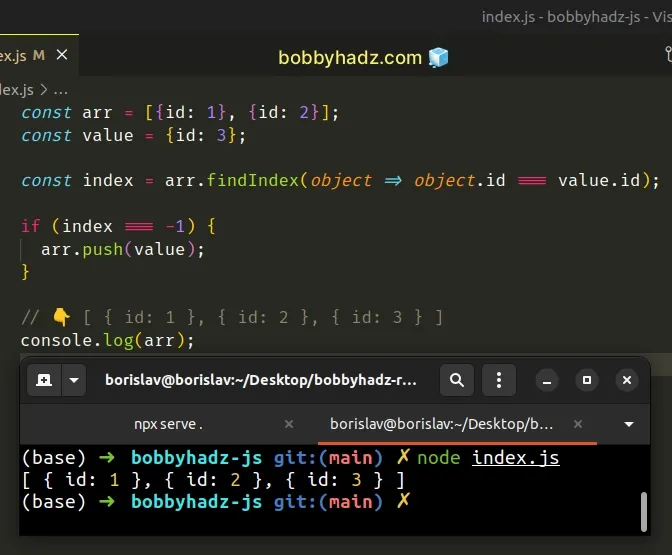The Array.findIndex method returns the index of the first element in the array that satisfies the supplied testing function.

The method returns `-1` if no elements satisfy the function.

In the example, we check if each element has an `id` property equal to a specific value.

index.js
```Copied!```const arr = [{id: 1}, {id: 2}];
const value = {id: 3};

const index = arr.findIndex(object => object.id === value.id);

console.log(index); // 👉️ -1
``````

The function we passed to the `Array.findIndex()` method gets called with each element in the array until it returns a truthy value or iterates over the entire array.

If the condition is never met, the `findIndex()` method returns `-1`, otherwise, it returns the index of the first element that meets the condition.
index.js
```Copied!```const arr = [{id: 1}, {id: 2}];
const value = {id: 3};

const index = arr.findIndex(object => object.id === value.id);
console.log(index); // 👉️ -1

if (index === -1) {
arr.push(value);
}

// 👇️ [{ id: 1 }, { id: 2 }, { id: 3 }]
console.log(arr);
``````

We check if the `findIndex()` method returned `-1` in the `if` statement.

If it did, we know that the value is not present in the array and we should add it.

You can also use the Array.indexOf method when working with primitives.

## #Array.push() Element if does not exist using Array.indexOf()

This is a three-step process:

1. Use the `Array.indexOf()` method to check if the value is contained in the array.
2. The `indexOf()` method returns `-1` if the value is not contained in the array.
3. If the returned index is `-1`, push the value into the array.
index.js
```Copied!```const arr1 = ['a', 'b', 'c', 'd'];
const value1 = 'e';

if (arr1.indexOf(value1) === -1) {
arr1.push(value1);
}

console.log(arr1); // 👉️ ['a','b','c','d','e']
``````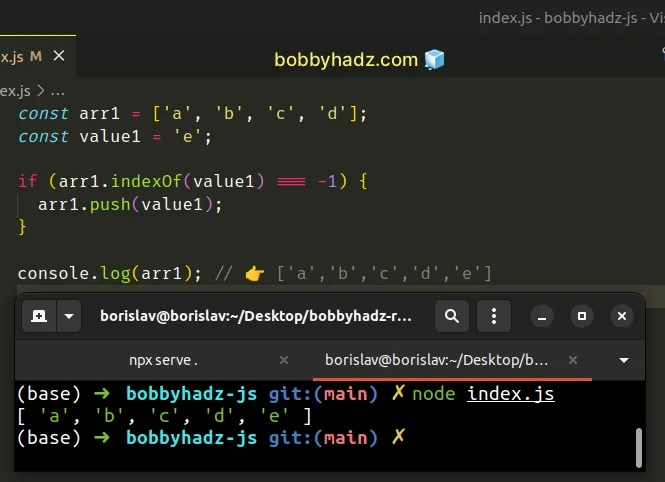We used the `Array.indexOf()` method instead of `Array.includes()`.

Note that this wouldn't work if the array contains objects. In this case we should use the `findIndex()` method from the previous subheading.

The Array.indexOf method returns the index of the first occurrence of the supplied value in the array.

If the value is not contained in the array, the method returns `-1`.

Our `if` statement checks if the value is not found in the array and adds it.

index.js
```Copied!```const arr = ['a', 'b', 'c', 'd'];
const value = 'e';

if (arr.indexOf(value) === -1) {
arr.push(value);
}

console.log(arr); // 👉️ ['a','b','c','d','e']
``````

I prefer using the `Array.includes()` method because it's easier to read and more intuitive.

I don't have to think about the implementation details of the method and whether it returns `-1` like `indexOf()`.

Alternatively, you can use the Array.find() method.

## #Array.push() Element if does not exist using Array.find()

This is a three-step process:

1. Use the `Array.find()` method to iterate over the array.
2. On each iteration, check if the specified value exists in the array.
3. If the `Array.find()` method returns `undefined`, push the value into the array.
index.js
```Copied!```const arr = [{id: 1}, {id: 2}];
const value = {id: 3};

const object = arr.find(object => {
return object.id === value.id;
});

console.log(object); // 👉️ undefined

if (object === undefined) {
arr.push(value);
}

// 👇️ [ { id: 1 }, { id: 2 }, { id: 3 } ]
console.log(arr);
``````

The function we passed to the `Array.find()` method gets called with each element (object) in the array.

On each iteration, we check if the current object has an `id` property with a value equal to the `id` property of the object to be added.

If the `Array.find()` method returns a truthy value, it short-circuits returning the matching array element.

If the condition is never met, the `Array.find()` method returns `undefined`.

Our `if` statement checks if the return value of the `Array.find()` method is equal to `undefined`.

If the condition is met, we push the object into the array.

## #Create an Array if it doesn't exist in JavaScript

To create an array if it doesn't exist:

1. Check if the type of the variable is equal to `undefined` or if the variable isn't an array.
2. If either of the conditions is met, initialize the variable to an array.
index.js
```Copied!```if (typeof arr === 'undefined' || !Array.isArray(arr)) {
var arr = [];
}

console.log(arr); // 👉️ [];
``````

We first check if the variable `arr` has a type of `undefined`.

The typeof operator doesn't throw an error even if the variable hasn't been declared.

If the variable `arr` has a type of `undefined`, we know that we have to create the array.

We used the logical OR `||` operator to add another condition.

If the code on the right-hand side of the operator runs, we know that the `arr` variable is declared.

In the second condition, we check if the variable is NOT of type `array`.

index.js
```Copied!```if (typeof arr === 'undefined' || !Array.isArray(arr)) {
var arr = [];
}

console.log(arr); // 👉️ [];
``````

If the variable is an array, we don't want to recreate it. However, if it isn't an array, we initialize it to one in the `if` block.

If either of the conditions evaluates to `true`, the `if` block runs and we declare the variable and set it to an empty array.

Note that we intentionally use the `var` keyword instead of the block-scoped `let` and `const`. This makes the `arr` variable accessible outside the `if` block.

The only way for this approach to fail is if you had previously declared the `arr` variable using `const`.

In this case, you wouldn't be allowed to redeclare an already declared variable.

If you had previously declared the variable using `let`, you can simply do the following check.

index.js
```Copied!```let arr;

if (!Array.isArray(arr)) {
arr = [];
}

console.log(arr); // 👉️ [];
``````

We know that the arr variable has been declared, so we don't have to use the `typeof` operator.

We also didn't use the `var` keyword. All we had to do is change the value of the `arr` variable if it isn't an array.

I wrote a book in which I share everything I know about how to become a better, more efficient programmer.You can use the search field on my Home Page to filter through all of my articles.